# Ceva theorem

(diff) ← Older revision | Latest revision (diff) | Newer revision → (diff)
A theorem on the relation between the lengths of certain lines intersecting a triangle. Let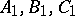be three points lying, respectively, on the sides,and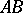of a triangle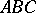. For the lines,andto intersect in a single point or to be all parallel it is necessary and sufficient that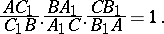Lines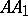,and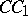that meet in a single point and pass through the vertices of a triangle are called Ceva, or Cevian, lines. Ceva's theorem is metrically dual to the Menelaus theorem. It is named after G. Ceva .
Ceva's theorem can be generalized to the case of a polygon. Let a point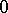be given in a planar polygon with an odd number of vertices, and suppose that the lines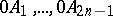intersect the sides of the polygon opposite torespectively in points,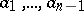. In this case